# Runge theorem

(diff) ← Older revision | Latest revision (diff) | Newer revision → (diff)

A theorem on the possibility of polynomially approximating holomorphic functions, first proved by C. Runge (1885) (cf. also Approximation of functions of a complex variable).

Letbe a simply-connected domain in the complex-plane. Then any functionholomorphic incan be approximated uniformly on compact sets insideby polynomials in. More precisely, for any compact set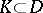andthere is a polynomial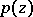with complex coefficients such thatfor all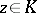.

In other words: Any functionholomorphic in a simply-connected domain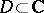can be represented as a series of polynomials inconverging absolutely and uniformly toon compact sets inside.

An equivalent statement of Runge's theorem: Letbe a compact set inwith connected complement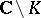; then any function holomorphic in a neighbourhood ofcan be approximated uniformly onby polynomials in. In this formulation, Runge's theorem is a special case of Mergelyan's theorem (cf. Mergelyan theorem).

The following theorem on rational approximation is also called Runge's theorem: Any functionholomorphic in a domaincan be uniformly approximated on compact sets insideby rational functions with poles outside.

Runge's theorem has many applications in the theory of functions of a complex variable and in functional analysis. A theorem analogous to Runge's theorem is valid for non-compact Riemann surfaces. An extension of Runge's theorem to functions of several complex variables is the Oka–Weil theorem (see Oka theorems).

How to Cite This Entry:
Runge theorem. Encyclopedia of Mathematics. URL: http://encyclopediaofmath.org/index.php?title=Runge_theorem&oldid=13069
This article was adapted from an original article by E.M. Chirka (originator), which appeared in Encyclopedia of Mathematics - ISBN 1402006098. See original article